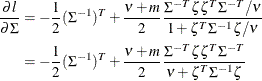The COPULA Procedure

Student’s t copula

Subsections:

Let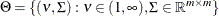and let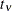be a univariate t distribution with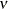degrees of freedom.

The Student’s t copula can be written as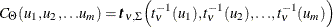where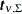is the multivariate Student’s t distribution with a correlation matrix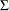withdegrees of freedom.

Simulation

The input parameters for the simulation are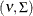. The t copula can be simulated by the following the two steps:

1. Generate a multivariate vector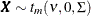following the centered t distribution withdegrees of freedom and correlation matrix.

2. Transform the vector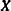into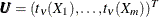, whereis the distribution function of univariate t distribution withdegrees of freedom.

To simulate centered multivariate t random variables, you can use the property thatif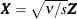, where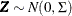and the univariate random variable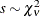.

Fitting

To fit a t copula is to estimate the covariance matrixand degrees of freedomfrom a given multivariate data set. Given a random sample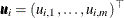,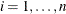that has uniform marginal distributions, the log likelihood is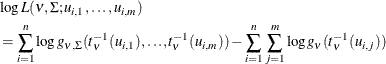wheredenotes the degrees of freedom of the t copula,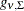denotes the joint density function of the centered multivariate t distribution with parameters,is the distribution function of a univariate t distribution withdegrees of freedom,is a correlation matrix, and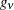is the density function of univariate t distribution withdegrees of freedom.

The log likelihood can be maximized with respect to the parameters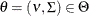using numerical optimization. If you allow the parameters into be such thatis symmetric and with ones on the diagonal, then the MLE estimate formight not be positive semidefinite. In that case, you need to apply the adjustment to convert the estimated matrix to positive semidefinite, as shown by McNeil, Frey, and Embrechts (2005), Algorithm 5.55.

When the dimension of the data m increases, the numerical optimization quickly becomes infeasible. It is common practice to estimate the correlation matrixby calibration using Kendall’s tau. Then, using this fixed, the single parametercan be estimated by MLE. By proposition 5.37 in McNeil, Frey, and Embrechts (2005),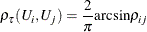where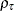is the Kendall’s tau and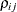is the off-diagonal elements of the correlation matrixof the t copula. Therefore, an estimate for the correlation is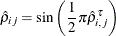where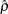and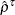are the estimates of the sample correlation matrix and Kendall’s tau, respectively. However, it is possible that the estimate of the correlation matrix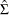is not positive definite. In this case, there is a standard procedure that uses the eigenvalue decomposition to transform the correlation matrix into one that is positive definite. Letbe a symmetric matrix with ones on the diagonal, with off-diagonal entries in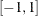. Ifis not positive semidefinite, use Algorithm 5.55 from McNeil, Frey, and Embrechts (2005):

1. Compute the eigenvalue decomposition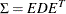, where
is a diagonal matrix that contains all the eigenvalues and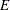is an orthogonal matrix that contains the eigenvectors.

2. Construct a diagonal matrix
by replacing all negative eigenvalues inby a small value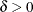.

3. Compute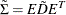, which is positive definite but not necessarily a correlation matrix.

4. Apply the normalizing operator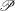on the matrix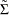to obtain the correlation matrix desired.

The log likelihood function and its gradient function for a single observation are listed as follows, where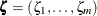, with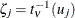, and g is the derivative of the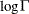function: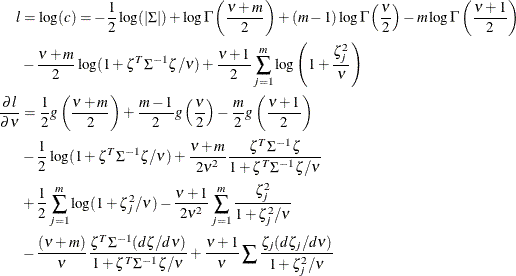The derivative of the likelihood with respect to the correlation matrix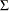follows: